top of page
Search

# What is K- Nearest Neighbors Algorithms In Machine Learning | Realcode4you

Before learn about K-Nearest Neighbors first we know about supervised and unsupervised machine learning algorithms.

Un-Supervised Learning

Organize a collection of unlabeled data items into categories .

• The instances are unlabelled and the goal is to organize a collection of data items into categories,

• The items within a category are more similar to each other than they are to items in the other categories.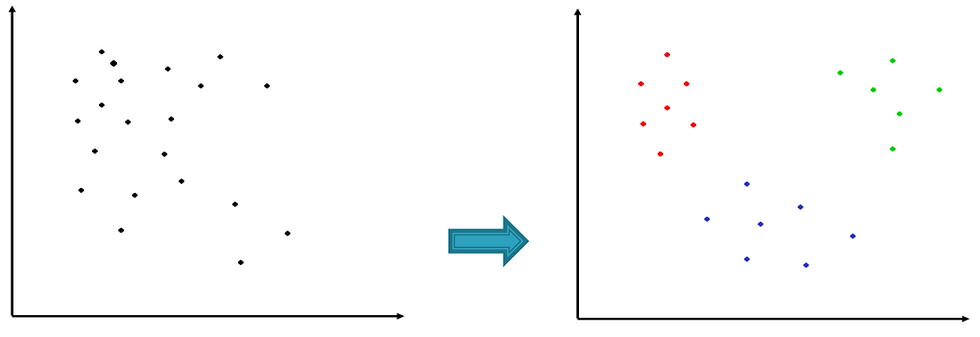Clustering is also good approach for anomaly detection.

Example: K-means

Supervised Learning

Predict the relationship between objects and class-labels (Hypothesis)

• Each object is labeled with a class.

• The target is to find the predictive relationship between objects and class-labels. (Hypothesis)Example:

• K-NN (K- Nearest Neighbor

• Decision Trees (Id3, C4.5)

• SVM (Support Vector Machines)

• ANN (Artificial Neural Network)

• NB (Naive Bayes)

K-Nearest-Neighbors Algorithm

• K nearest neighbors (KNN) is a simple algorithm that stores all available cases and classifies new cases based on a similarity measure (distance function)

• KNN has been used in statistical estimation and pattern recognition since 1970’s

• A case is classified by a majority voting of its neighbors, with the case being assigned to the class most common among its K nearest neighbors measured by a distance function.

• If K=1, then the case is simply assigned to the class of its nearest neighbor

Features

• All instances correspond to points in an n-dimensional Euclidean space

• Classification is delayed till a new instance arrives

• Classification done by comparing feature vectors of the different points

• Target function may be discrete or real-valued

-Instance based learning algorithm

- Lazy learner: needs more computation time during

classification

- Conceptually close to human intuition: e.g., people with

similar income would live in the same neighborhood

Classification strategy:

K-NN assigns the instance to relative class group by identifying the most frequent class label.

In some case when numeric instances are involved proximity distance measures is required. E.g., Euclidean Distance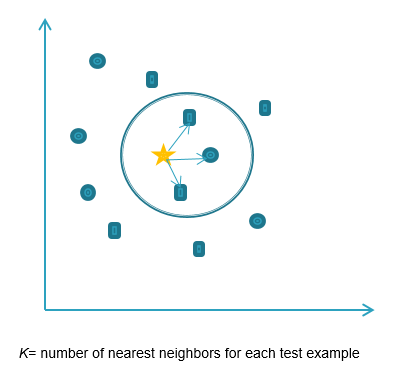KNN Example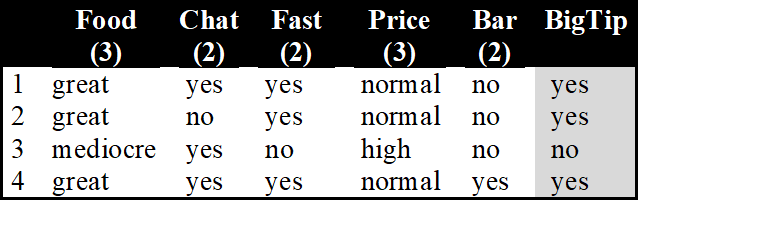Similarity metric: Number of matching attributes (k=2)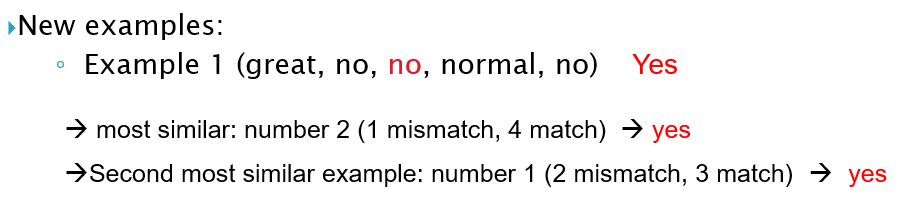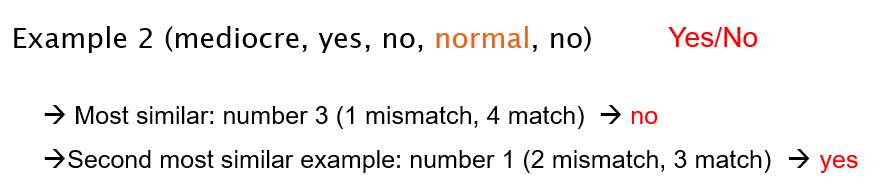Selecting the Number of Neighbors

-Increase k:

• Makes KNN less sensitive to noise

- Decrease k:

• Allows capturing finer structure of space

- Pick k not too large, but not too small (depends on data)

1. Need distance/similarity measure and attributes that “match” target function.

2. For large training sets,

• Must make a pass through the entire dataset for each classification. This can be prohibitive for large data sets.

3. Prediction accuracy can quickly degrade when number of attributes grows.

Using K-NN in R

Case study: Iris data set

```df <- data(iris)

# look into data structure
str(iris)
dim(iris)```

Generate a random sample of all data

```# Generate a random sample of all data
# in this case 82% of the  dataset.
randSelection <- sample(1:nrow(iris), 0.82 * nrow(iris))
randSelection```

Normalization

```# data normalization f
normalization <-function(x) { (x -min(x))/(max(x)-min(x))  }

# Run nomalization on on coulumns which are the predictors

irisNormalized <- as.data.frame(lapply(iris[,c(1:4)], normalization))

summary(irisNormalized)```

Training & Testing

```## seperate data into training and testing to #check model accuracy
#  get training data
training <- irisNormalized[randSelection,]
nrow(training)

# get testing data
testing <- irisNormalized[-randSelection,]
nrow(testing)```

Obtain the class label

```# obtain the class label of train dataset because as it will
#be used as argument in knn classifier
targertClass <- iris[randSelection,5]
targertClass
summary(targertClass)

# extract 5th column if test dataset to measure the
#accuracy
testClass <- iris[-randSelection,5]
summary(testClass)```

Install package class for k-nn & Build the model

```library(class)
# building the model for classification
# run knn classifier
# here we use k = 10

classificationModel <-  knn(training,testing,cl=targertClass,k=10)
classificationModel

```

Confusion matrix

```#create confusion matrix to check model
# performance
ConfMatrix <- table(classificationModel,testClass)
ConfMatrix```

OUTPUT:Model Accuracy

```#Calculate model accuracy
modelAccuracy <- function(x){sum(diag(x)/(sum(rowSums(x)))) * 100}
modelAccuracy(ConfMatrix)```

To get help in K- Nearest Neighbors Algorithms or other machine learning algorithms you can contact us or directly send your assignment requirement details at:

realcode4you@gmail.com